# allrite

## White Dwarfs & Neutron Stars – Superfluidity

The high densities of matter internal to a neutron star allow another interesting phenomenon to occur, superfluidity. Roughly speaking, superfluids have the property of experiencing no friction. It is a quantum mechanical effect, and for neutron stars is related to the same mechanism as produces superconductors.

Superfluidity relies on the wave-particle duality of quantum mechanics. To gain an idea of why superfluids can exist in neutron stars, consider the non-relativistic de Broglie relation for the wavelength,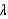, of a particle of mass m and velocity v.The kinetic energies, and mean thermal energies of a non-relativistic and monatomic ideal gas are respectively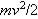and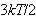, where k is again Boltzmann’s constant. Combining these three equations, we can find the temperature at which the wave-like behaviour of particles to show.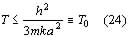Now, a is related to the spacing between particles. Normally, this temperature is of the order of a fraction of a degree kelvin for particles heavier than an electron, and indeed has only been observed in liquid helium. However, the extremely dense nature of neutron star material allows leads to a correspondingly high T0, or degeneracy temperature as it known. This allows quantum effects to come into play.

There are two categories of quantum particles. Fermions, mentioned earlier, include neutrons, protons and electrons, have spins equal to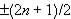(all the fermions mention actually have spin 1/2). The other types are bosons, such as photons and neutrinos, which possess integer spins. The same type of classifications can also be applied to atoms and molecules. For instance, the helium-4 isotope has 2 electrons, 2 protons, and 2 neutrons, each of the corresponding particles possessing spins of the opposite sign. Consequently, the total spin for the system is zero, it is a boson. Helium-3, on the other hand has a half-integer total spin, so is a fermion. The type of particle determines whether the system obeys Bose or Fermi statistics, but an understanding of these is not important to the discussion. What is important is that as the temperatures of systems fall towards the degeneracy temperature, the particles cease behaving classically, and now start to obey the previously mentioned statistics.

There is a certain transition temperature at which, for bosonic systems, Bose condensation occurs. What this means is that all the “particles” (eg atoms, not true bosons like photons however) in the system occupy a single quantum state. What this means is that all the particles do exactly the same thing at the same time. This gives the whole system a de Broglie “wave” that, in a confined system, can only take on discrete wavelengths. This large scale quantum correlation between particles is basically what causes superfluidity.

However, this does not explain how neutrons in a neutron star can be superfluid, because the single neutrons are fermions. The solution to this problem derives from the study of superconductivity. Electrons flowing through a conductor form what are known as Cooper pairs. These pairs will have a net spin of zero, and so are bosons. At a critical temperature, these undergo bose condensation and the resistance in the material disappears. Similarly neutrons will form Cooper pairs, of spin +/- 1, and the system will become bosonic, and so below the degeneracy temperature the neutrons will undergo Bose condensation and become superfluid. Also, any protons remaining will form Cooper pairs, and like electrons (only now with a positive charge) they become superconducting.